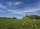# Pythagorean theorem - high school - math problems

1. Triangular pyramidWhat is the volume of a regular triangular pyramid with a side 3 cm long?
2. KLM triangleFind the length of the sides of the triangle KLM if m = 5cm height to m = 4.5 cm and size MKL angle is 70 degrees.
3. Rectangular fieldA rectangular field has a diagonal of length 169m. If the length and width are in the ratio 12:5. Find the dimensions of the field, the perimeter of the field and the area of the field.
4. Tangent spheresA sphere with a radius of 1 m is placed in the corner of the room. What is the largest sphere size that fits into the corner behind it? Additional info: Two spheres are placed in a corner of a room. The spheres are each tangent to the walls and floor an
5. Vertices of a right triangleShow that the points D(2,1), E(4,0), F(5,7) are vertices of a right triangle.
6. Axial cut of a rectangleCalculate the volume and surface of the cylinder whose axial cut is a rectangle 15 cm wide with a diagonal of 25 cm long.
7. Circle describedThe radius of the circle described to the right triangle with 6 cm long leg is 5 cm. Calculate the circumference of this triangle.
8. Infinite sum of areasAbove the height of the equilateral triangle ABC is constructed an equilateral triangle A1, B1, C1, of the height of the equilateral triangle built A2, B2, C2, and so on. The procedure is repeated continuously. What is the total sum of the areas of all tri
9. Diamond diagonalsFind the diamond diagonal's lengths if the area is 156 cm2 and side is 13 cm long.
10. Diagonals of a rhombus 2One diagonal of a rhombus is greater than other by 4 cm . If the area of the rhombus is 96 cm2, find the side of the rhombus.
11. Diagonals of the rhombusHow long are the diagonals e, f in the diamond, if its side is 5 cm long and its area is 20 cm2?
12. Rectangular trapezoidThe ABCD rectangular trapezoid with the AB and CD bases is divided by the diagonal AC into two equilateral rectangular triangles. The length of the diagonal AC is 62cm. Calculate trapezium area in cm square and calculate how many differs perimeters of the.
13. Rectangular gardenThe sides of the rectangular garden are in ratio 1: 2. The diagonal has a length of 20 meters. Calculate the area and perimeter of the garden.
14. Right 24Right isosceles triangle has an altitude x drawn from the right angle to the hypotenuse dividing it into 2 unequal segments. The length of one segment is 5 cm. What is the area of the triangle? Thank you.
15. Right isosceles triangleRight isosceles triangle has an altitude x drawn from the right angle to the hypotenuse dividing it into 2 equal segments. The length of one segment is 5 cm. What is the area of the triangle?
16. Three pointsThree points A (-3;-5) B (9;-10) and C (2;k) . AB=AC What is value of k?
17. On lineOn line p: x = 4 + t, y = 3 + 2t, t is R, find point C, which has the same distance from points A [1,2] and B [-1,0].
18. Center of line segmentCalculate the distance of the point X [1,3] from the center of the line segment x = 2-6t, y = 1-4t ; t is .
19. Distance problem 2A=(x,2x) B=(2x,1) Distance AB=√2, find value of x
20. Distance problemA=(x, x) B=(1,4) Distance AB=√5, find x;

Do you have an interesting mathematical word problem that you can't solve it? Enter it, and we can try to solve it.

We will send a solution to your e-mail address. Solved examples are also published here. Please enter the e-mail correctly and check whether you don't have a full mailbox.

Please do not submit problems from current active competitions such as Mathematical Olympiad, correspondence seminars etc...

Pythagorean theorem is the base for the right triangle calculator.